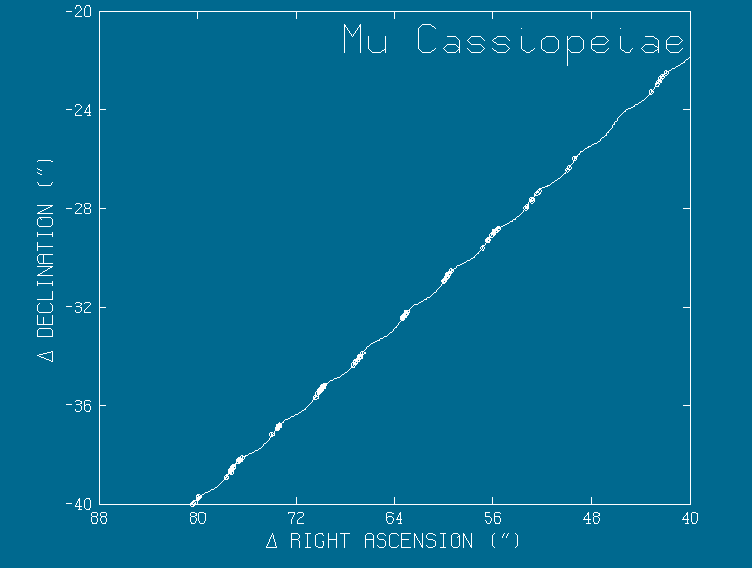## Mu Cassiopeiae

This plot shows a few selected points of astrometric data supplied by Sara Lee Lippincott of the Population II binary Mu Cas. The complete data set was used by the Binary program along with speckle data and spectroscopic data to simultaneously solve for the elements of the orbit and the parallax and proper motion.

An example of the output (not the ultimate solution) is:

``` The X and Y centers are for the epoch 2000.0000
Period=                                         21.56053487     +-0.3244249E-01
Time of periastron passage=                     1975.740511     +-0.5210147E-01
Eccentricity=                                  0.5821179865     +-0.7898859E-02
Angle of line of nodes=                         46.45821624     +-0.5948454
Inclination=                                   -111.0684255     +-0.5165805
Angle from node to periastron=                  333.3541076     +- 1.374378
Semimajor axis in "=                           0.1836691371     +-0.2047917E-02
Ratio of semimajor axes (total to primary)=     5.438056031     +-0.8116256E-01
Parallax=                                      0.1291892022     +-0.1723935E-02
X center=                                       159.8149273     +-0.1509620E-01
X proper motion=                                3.424510504     +-0.8237344E-03
X proper motion acceleration=                  0.5962608941E-05 +-0.1048381E-04
Y center=                                      -77.00214119     +-0.1418460E-01
Y proper motion=                               -1.589135992     +-0.8027955E-03
Y proper motion acceleration=                  -.4975650962E-04 +-0.1030322E-04